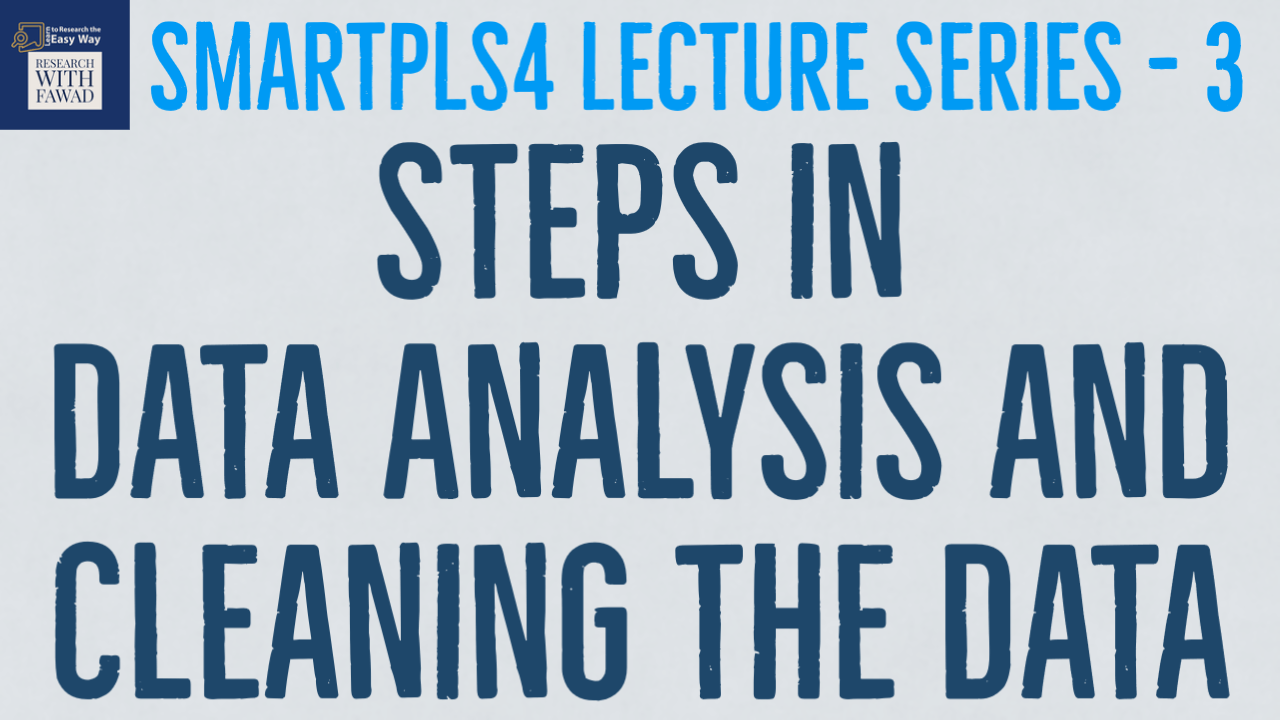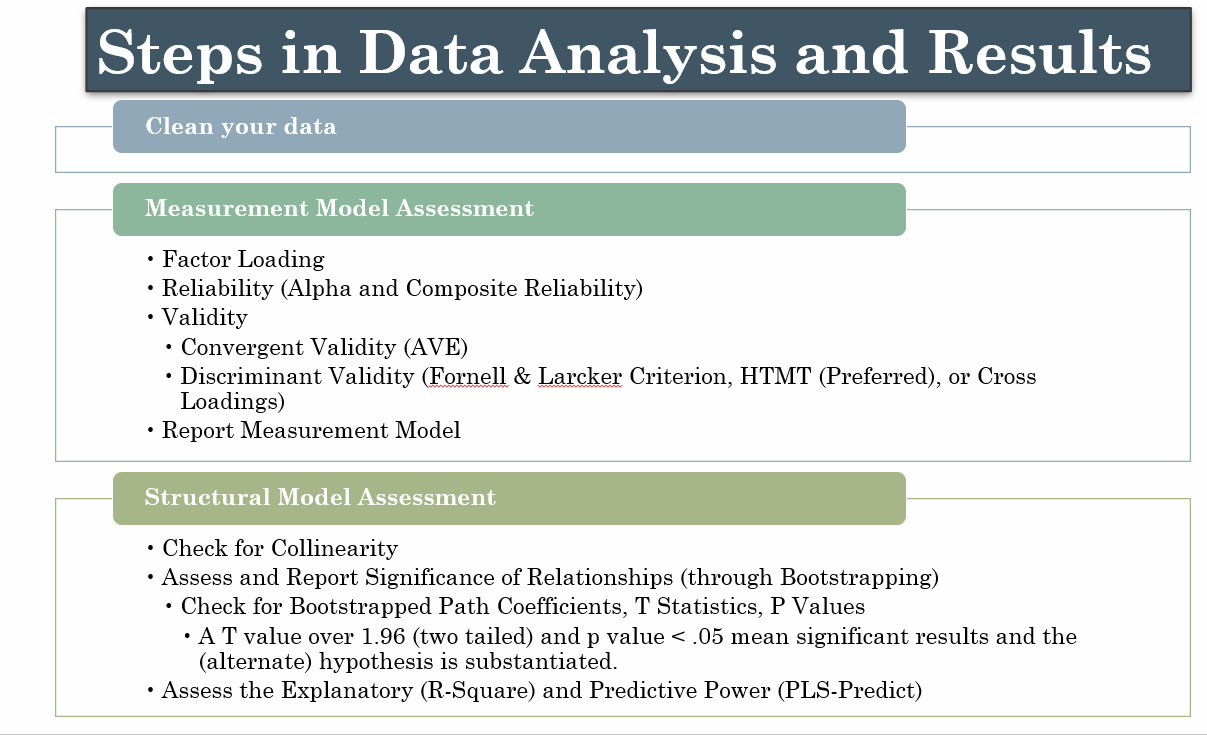# Steps in Data Analysis - SmartPLS4 Series

Learn the steps in Data Analysis when using Structural Equation Modelling.### The Process of Data Analysis

The focus of the session is to help scholars learn the basic and advance techniques in Data Analysis when using SmartPLS4.

Click Here to visit the Complete Playlist

## Steps in Data Analysis

The first stage in a data analysis process using SmartPLS is data cleaning, focused on handling missing values, outliers, and data quality concerns through respondent misconduct to ensure a valid dataset. The next step is Measurement Model Assessment, which involves assessing the quality of the  measurement indicators in capturing the underlying constructs. Examining factor loadings, reliability using metrics such as Cronbach’s alpha and composite reliability, and validating the constructs using convergent and discriminant validity tests.
The Structural Model Assessment is the next step following the measurement model assessment.  In this step, the links between latent constructs are assessed. The relationship is assessed via bootstrapping, to determine path coefficients, t-statistics, and p-values. A t-value more than 1.96 and a p-value less than 0.05 usually imply statistically significant results. Finally, you assess your structural model’s explanatory strength (R-square) and predictive ability (PLS-Predict) for making out-of-sample predictions.

Following are the steps to follow for data analysis using SmartPLS4
• Measurement Model Assessment
• Reliability (Alpha and Composite Reliability)
• Validity
• Convergent Validity (AVE)
• Discriminant Validity (Fornell & Larcker Criterion, HTMT (Preferred), or Cross Loadings)
• Report Measurement Model
• Structural Model Assessment
• Check for Collinearity
• Assess and Report Significance of Relationships (through Bootstrapping)
• Check for Bootstrapped Path Coefficients, T Statistics, P Values
• A T value over 1.96 (two tailed) and p value < .05 mean significant results and the (alternate) hypothesis is substantiated.
• Assess the Explanatory (R-Square) and Predictive Power (PLS-Predict)## Videos for Each Step in Data Analysis

• Step by Step Approach to Data Analysis using Structural Equation Modelling (See Description). The short session guides on the steps for data analysis when using Structural Equation Modelling.

Data Cleaning
https://youtu.be/dZdIiEsgHWE